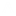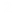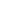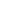In my previous post, I talked about the exploration/exploitation dilemma in optimization, and then defined an algorithm that seeks to resolve it. The algorithm described in that post was a variation of neighborhood search and hill climbing methods. The amount of time it spends looking for improvements in the “neighborhood” of a given solution is a direct function of the magnitude of improvement that a current best solution demonstrates over the previous best.

Large improvements cause the algorithm to remain in the current best solution’s neighborhood for significant lengths of time. While this is a good thing if the neighborhood is rich in potential improvements, it presents a problem if the neighborhood turns out to be sparse in improving solutions.

To counter this, I outline another, far more exploratory, numerical optimization algorithm. This algorithm symetrically interleaves its exploration and exploitation steps, dividing its time equally betweem searching the neighborhood of the current global best, and searching the global solution space in a purely random pattern.

After initially evaluating a solution at random and setting its score as the global best, the algorithm evaluates another solution in the global best’s neighborhood. If this solution’s score is better than that of the global best, it becomes the new global best, otherwise the optimizer tries a protential solution chosen at random from the entire search space. If this solution is better than global best, it becomes the global best and the search resumes from its neighborhood, otherwise the search returns to the current global best’s neighborhood to try another solution at random.

In this way, on every other step, the algorithm bounces between searching the whole space randomly and a much more focussed search in the global best’s neighborhood.

The algorithm was designed to optimize machine learning model parameters, but it can be applied to any numerical optimization problem.

Below is an outline of the algorithm, and below that is a Python implementation of the algorithm tuning the parameters of a LightGBM prediction model.

### Interleaved Neighborhood Algorithm

``````
evaluate random solution rs

set var global_best to rs score
set var best_parameters to rs parameters
set var horizon to "local"

while true do

if horizon = "local"

neighborhood solution ns = gaussian distr(mean = best_parameters, deviation = random(0.1...1.0))

if ns better than global_best

set var global_best to ns score
set var best_parameters to ns parameters
continue

else

set var horizon to "global"
coninue

else

evaluate global random solution gs

if gs score better than global_best

set var global_best to gs score
set var best_parameters to gs parameters
set var horizon to "local"
continue

else:

set var horizon to "local"
continue

``````

### Optimizing A LightGBM Machine Learning Model

The Python code below uses the Interleaved Neighborhood Algorithm to search through the space of parameters of a LightGBM machine learning model. The model is trained on, and used to make predictions about, the Kaggle Iowa housing dataset.

LightGBM is a gradient boosting framework with a large number of parameters, each of which can take a huge range of values. This makes parameter optimization a nontrivial problem. For simplicity, the demonstration below uses only a few of the dozens of model parameters available for tuning.

temp.py

```   1
2     import numpy as np
3     import pandas as pd
4     from lightgbm import LGBMRegressor
5     from sklearn.metrics import mean_absolute_error
6     from sklearn.model_selection import train_test_split
7     from random import randrange, uniform, gauss
8     import clevercsv
9
10
13         "train.csv",
14         encoding = "UTF-8",
15         index_col = "Id")
16
18         "test.csv",
19         encoding = "UTF-8",
20         index_col = "Id")
21
22
23     #Seperate predictors from target variable
24     X = complete_train.drop(
25         columns = "SalePrice")
26
27     y = complete_train[
28         "SalePrice"]
29
30
31     #Encode categoricals and impute missing data
32     def encode_impute(*datasets):
33         for dataset in datasets:
34             for column in dataset.columns:
35                 dataset[
36                     column].fillna(
37                     -999,
38                     inplace = True)
39                 if dataset[
40                     column].dtype ==  "object":
41                     dataset[
42                         column] = dataset[
43                         column].astype("category", copy = False)
44
45     encode_impute(X)
46
47
48     #Create validation set
49     X_train, X_valid, y_train, y_valid = train_test_split(X, y)
50
51
52     #Model evaluation
53     def model_check(parameters):
54
55         local_copy ={
56             key:value for key,value in parameters.items()}
57
58         model = LGBMRegressor().set_params(**local_copy)
59         model.fit(X_train, y_train)
60         prediction = model.predict(X_valid)
61         error_rate = mean_absolute_error(y_valid, prediction)
62
63         return {"score": error_rate, "parameters": parameters}
64
65
66     #Parameter generation
67     def param_gen():
68
69         k = randrange(10000)
70
71         parameters = {
72            "boosting_type": "dart",
73            "n_estimators": k,
74            "num_leaves": randrange(round(k/3 * 2)),
75            "learning_rate": uniform(0.01, 1)}
76
77         return parameters
78
79
80
81     #square_neighborhood algorithm
82     def interleaved_neighborhood():
83
84         initialize = model_check(param_gen())
85
86         global_best = {
87             "score": initialize["score"],
88             "parameters": initialize["parameters"]}
89
90         with open(
91             "values.csv", "a", encoding = "UTF-8") as valuefile:
92             values = clevercsv.writer(valuefile)
93             values.writerow(
94                 [global_best["score"],
95                 global_best["parameters"]])
96
97         horizon = "local"
98
99
100         while True:
101
102             try:
103
104                 if horizon == "local":
105
106                     perturb = {
107                         key:abs(gauss(value, uniform(0.1, 2.0)))
108                         if type(value) != str
109                         else value for key,value
110                         in global_best["parameters"].items()}
111
112                     rounded = {
113                         key:round(value)
114                         if key in ["n_estimators", "num_leaves"]
115                         else value for key,value in perturb.items()}
116
117                     local_solution = model_check(rounded)
118
119                     if local_solution["score"] < global_best["score"]:
120
121                         with open(
122                             "values.csv", "a", encoding = "UTF-8") as valuefile:
123                             values = clevercsv.writer(valuefile)
124                             values.writerow(
125                                 [local_solution["score"],
126                                 local_solution["parameters"]])
127
128                         global_best["score"] = local_solution["score"]
129                         global_best["parameters"] = local_solution["parameters"]
130
131                         continue
132
133
134                     else:
135
136                         horizon = "global"
137
138                         continue
139
140
141                 else:
142
143                     random_solution = model_check(param_gen())
144
145                     if random_solution["score"] < global_best["score"]:
146
147                         with open(
148                             "values.csv", "a", encoding = "UTF-8") as valuefile:
149                             values = clevercsv.writer(valuefile)
150                             values.writerow(
151                                 [random_solution["score"],
152                                 random_solution["parameters"]])
153
154                         global_best["score"] = random_solution["score"]
155                         global_best["parameters"] = random_solution["parameters"]
156
157                         horizon = "local"
158
159                         continue
160
161                     else:
162
163                         horizon = "local"
164
165                         continue
166
167
168
169             except Exception as error:
170
171                 print(error)
172
173
174
175
176
177     interleaved_neighborhood()
178
179
180
181
```Full code repo available on Github

Related posts:

Square Neighborhood Algorithm: Balancing Exploration And Exploitation In Optimization

Speculative Fiction Bot

EXP-RTL: Exponential Retaliation In Iterated Prisoner’s Dilemma Games